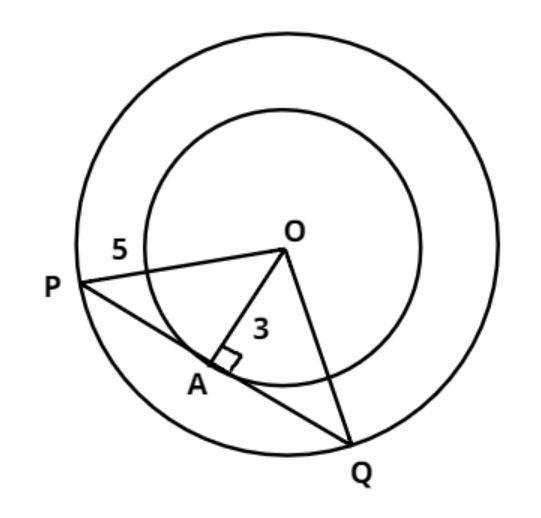Courses
Courses for Kids
Free study material
Free LIVE classes
MoreLIVE
Join Vedantu’s FREE Mastercalss

# Two concentric circles of radii 5cm and 3cm. Find the length of the chord of the larger circle which touches the smaller circle.Verified
264.6k+ views
Hint: - Perpendicular drawn from the center to the chord of the circle bisects the chord.Two concentric circles of radii 5cm and 3cm are shown in figure.
$\Rightarrow {\text{OA = 3cm and OP = 5cm}}$
Now we have to find out the chord of the larger circle which touches the smaller circle.
$\Rightarrow$So, from the figure we have to find out the value of PQ.
In triangle OAP by, Pythagoras Theorem, we have
${\text{O}}{{\text{A}}^2}{\text{ + A}}{{\text{P}}^2}{\text{ = O}}{{\text{P}}^2} \\ \Rightarrow {\text{A}}{{\text{P}}^2} = {5^2} - {3^2} \\ \Rightarrow {\text{A}}{{\text{P}}^2} = 25 - 9 = 16 = {4^2} \\ \Rightarrow {\text{AP = 4cm}} \\$
As we know that the perpendicular drawn from the center to the chord of the circle bisects the chord.
$\therefore {\text{PQ = 2AP = 2}} \times 4 = 8cm$
So, the length of the chord which touches the smaller circle is 8 cm.

Note: - Concentric circles are the circles with a common center and whenever we face such types of problem first draw the pictorial representation then draw the perpendicular from the center on the chord of the circle which divide the chord into two equal parts, then apply Pythagoras Theorem we will get the required answer.
Last updated date: 01st Oct 2023
Total views: 264.6k
Views today: 4.64k